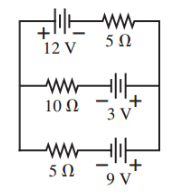# Problem: What is the current through the 10 Ω resistor in the figure?  Express your answer to two significant figures and include the appropriate units. Is the current from left to right or right to left?a) left to right b) right to left

###### FREE Expert Solution

Call currents i1, i2, i3 from top branch to bottom. Assume i1 goes right to left, i2 goes left to right, i3 goes left to right. Kirchoff's junction equation:

i1 = i2 + i3

Using two counterclockwise current loops (top and bottom), write loop equations starting at top left corner of each loop.

Top loop:

−5i1 + 12 − 10i2 + 3 = 0

Bottom loop:

−3 + 10i2 − 5i3 + 9 = 0

79% (172 ratings)###### Problem Details

What is the current through the 10 Ω resistor in the figure?  Express your answer to two significant figures and include the appropriate units.

Is the current from left to right or right to left?

a) left to right

b) right to left